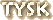# Mental Flexibility Test

Sent to me by a fiendish friend, I found this both fun and challenging. If anyone knows the source of this, please drop me a note. Also, I'd like to find more like it.This test does not measure your intelligence, your fluency with words, and certainly not your mathematical ability. It will, however, give you some gauge of your mental flexibility and creativity. It has been found that few people could solve more than half on the first day. Many reported getting answers long after the test had been set aside, at unexpected moments when their minds were relaxed; and some reported solving questions over a period of several days.

Take this as a personal challenge.

Example: 16 = O in a P Answer: 16 = ounces in a pound

1. 26 = L of the A

2. 7 = D of the W

3. 1001 = A N

4. 12 = S of the Z

5. 54 = C in a D (with J)

6. 9 = P in the S S

7. 88 = PK

8. 13 = S on the A F

9. 32 = D F at which W F

10. 18 = H on a G C

11. 90 = D in a R A

12. 200 = D for P G in M

13. 8 = S on a S S

14. 3 = B M (S H T R)

15. 4 = Q in a G

16. 24 = H in a D

17. 1 = W on a U

18. 5 = D in Z C

19. 57 = H V

20. 11 = P on a F B T

21. 1000 W that a P is W

22. 29 = D in F in a L Y

23. 64 = S on a C B

24. 40 = D and N of the G F

25. 76 = T in the BP

26. 50 = W to L Y L

27. 99 = B of B on the W

28. 60 = S in a M

29. 1 = H on a U

30. 9 = J on the S C

31. 7 = B for S B

32. 21 = D on a D

33. 7 = W of the A W

34. 15 = M on a D M C

Scoring:

1-6 Questions   = Average

6-12 Questions  = Somewhat Intelligent

12-18 Questions = Intelligent

18+ Questions   = Genius!

 Answers? Sure, I got 'em. Just send an While you're at it, send along any comments or suggestions you may have for this site.

Had enough or, do you want more?
Go ahead – take a chance on the
Mental Flexibility Test IIwww.tysknews.com

revised: 26 feb 2005